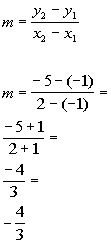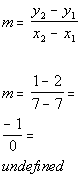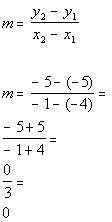# College Algebra Tutorial 25

College Algebra
Tutorial 25: Slope of a LineAnswer/Discussion to 1a

(2, -5) and (-1, -1)*Plug in x and y values into slope formula

*Simplify

Make sure that you are careful when one of your values is negative and you have to subtract it as we did in line 2.  -5 - (-1) is not the same as -5 - 1 and 2 - (-1) is not the same as 2 - 1.

The slope of the line is -4/3.

Since the slope is negative, the line would fall (left to right).Answer/Discussion to 1b

(7, 1) and (7, 2)*Plug in x and y values into slope formula

*Simplify

The slope of the line is undefined.

Since the slope is undefined, the line is vertical.Answer/Discussion to 1c

(1, 7) and (-2, -2)*Plug in x and y values into slope formula

*Simplify

Make sure that you are careful when one of your values is negative and you have to subtract it as we did in line 2.  7 - (-2) is not the same as 7 - 2 and 1 - (-2) is not the same as 1 - 2.

The slope of the line is 3.

Since the slope is positive, the line would rise (left to right).Answer/Discussion to 1d

(-1, -5) and (-4, -5)*Plug in x and y values into slope formula

*Simplify

Make sure that you are careful when one of your values is negative and you have to subtract it as we did in line 2.  -5 - (-5) is not the same as -5 - 5 and -1 - (-4) is not the same as -1 - 4.

The slope of the line is 0.

Since the slope is zero, the line is horizontal.